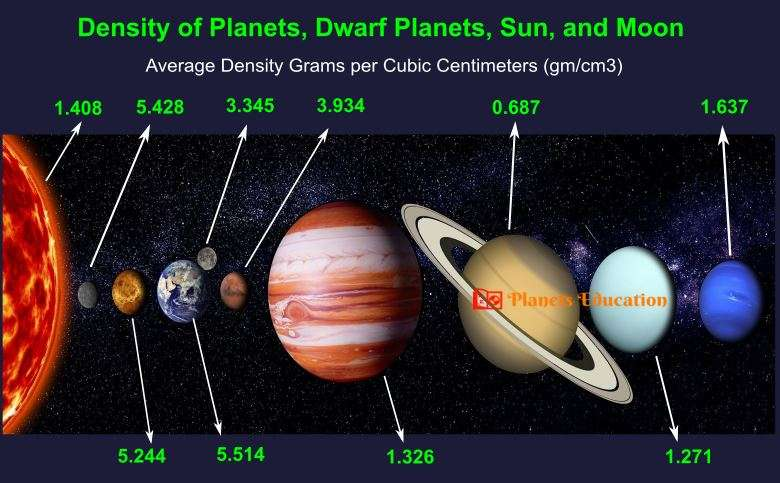# Density of All Planets in Solar System With FactsTo measure the density of planets all you need to know the mass and radius of planets. From the radius, the volume of the planet would be known. Now the planet’s density would be the “Mass divided by Volume” (M/V).

One of the facts about Planets Density is, it is variable throughout its radius. It means, most of the planets have layers and the density of all layers would be different. Such as, the Earth has 3 layers, Crust, Mantle, and Core. And each layer’s density is different. In this way, the density of all planets is different throughout their depth (radius). So, here we have mentioned, the average or mean density of all planets. You may find some other facts related to the highest, lowest, and average density of any particular planet.

But, here you can also find some interesting facts about the density of planets. Such as, which planet is the densest, or least dense planet, or the planet less dense than water, or which planet can float on water, etc.

As we have mentioned, we need to know the Mass and Volume to determine Planets Density. So, here in one of our articles, we have explained: “how do scientists measure the mass of a planet”. You should check it out.

## The Density of Planets

Below, you can check the density of all eight planets. The mentioned density is in the unit of Grams per Cubic Centimeters (gm/cm3). The average density of planets in order are:- Earth, Mercury, Venus, Mars, Neptune, Jupiter, Uranus, and Saturn.

For reference (1 gm/cm3 = 1000 kg/m3). The density of water is almost 1 gm/cm3 or 997 kg/m3.

### Density of Mercury

5.428 gm/cm3
Mercury is the second densest planet of our solar system after the Earth (5.514 gm/cm3). If we do not consider gravitational compression for both planets then Mercury would be denser than earth. Without considering gravitational compression the Mercury’s density would be 5.3 gm/cm3 while the earth’s density would be around 4.4 gm/cm3. (Gravitational Compression – is a phenomenon in which gravity compresses the object and increases its density while reducing the object’s size.)

### Density of Venus

5.244 gm/cm3
Venus is the third densest in the solar system planets. Though it has one of the densest atmospheres with around 92 times of the earth.

### Density of Earth

5.514 gm/cm3
Our Earth is the densest planet in the solar system. Though its density increases with depth. The Crust density is almost 2.5-3.0 gm/cm3, for Mantle 3.0-3.5 gm/cm3, and the inner core density is approximate 13 gm/cm3. So the mean density of the earth is 5.514 gm/cm3.

### Density of Mars

3.934 gm/cm3
Mars is the least dense terrestrial planet. Though it has more density in comparison to giant planets. Its atmosphere density is also lower, and the highest atmospheric density on Mars is almost the same as that found 32 km above the earth’s surface.

### Density of Jupiter

1.326 gm/cm3
Planet Jupiter is the 2nd densest giant-planet after Neptune. It is the largest planet but made of gases, so the density of this planet is lower.

### Density of Saturn

0.687 gm/cm3
Saturn is the least dense planet in our solar system. The density of Saturn is just 0.687 gm/cm3, which is less than the density of water (1 gm/cm3). So, Planet Saturn can float on water because of its lower density than water.

### Density of Uranus

1.271 gm/cm3
Uranus is the 2nd least dense planet of our solar system after Saturn. It is bigger than planet Neptune in size but due to its lower density, the mass of this planet is comparatively low.

### Density of Neptune

1.637 gm/cm3
Neptune is the densest giant planet but has a low density compared to all terrestrial planets. The size of this planet is smaller than Uranus, but it more massive compared to Uranus. This fact (higher mass than Uranus) happens because of its higher density.

## The Density of Dwarf Planets

Below, you can find the density of five dwarf planets. And here also, the mentioned density is in the unit of Grams per Cubic Centimeters (gm/cm3). (FYI – The dwarf planet’s density given here is the average and also not confirmed properly by scientists, they have the range).

 Ceres Density 2.162 gm/cm3 It is the 2nd densest dwarf planet. Though it has low density, and some scientists believe it may contain 10% porosity at the core.

 Makemake Density 1.712 gm/cm3 It has a very low density of just 1.712 gm/cm3 and is the lowest density in all dwarf planets. But according to another method (because of adopting different sizes), the density of Makemake is almost 2.1 gm/cm3.

 Haumea Density 2.012 gm/cm3 Haumea’s mean density is 2.01 gm/cm3.

 Eris Density 2.431 gm/cm3 Eris is the densest dwarf planet.

 Pluto Density 1.855 gm/cm3 The mean density of Pluto is just 1.85 gm/cm3.

## The density of the Sun and Moon

Now, below you can find the density of the Sun and Moon.

 Density of Sun 1.408 gm/cm3 The average density of the sun is very less (1.408 gm/cm3). Compare to the earth’s average density (5.514 gm/cm3) it just 0.255 times. But the center density of the sun is 162.2 gm/cm3, which is almost 12.4 times of earth’s center density (13.1 gm/cm3).

 Density of Moon 3.345 gm/cm3 This is the average density of the moon and it is almost 60.5% of the earth’s average density.

Now, you have got to know the density of all planets, dwarf planets, the sun, and the moon with some interesting facts. You should also check below some other facts related to the planets.